Note: this page offers miscellaneous, possibly evolving, information intended to support the emergence of insight regarding a particular topic. It’s a resource to support my own thinking, and may or may not be useful to others.

## Wavelength vs. Wavenumber / Frequency

Note that “wavenumber” is just frequency divided by the speed of light. Sometimes I may write “frequency” but offer a wavenumber. Wavenumber might be considered to be an alternative unit for expressing frequency.

## Earth’s emissions at TOA

As a reference, a sample spectrum of Earth’s emissions to space is depicted below (Schmidt 2010).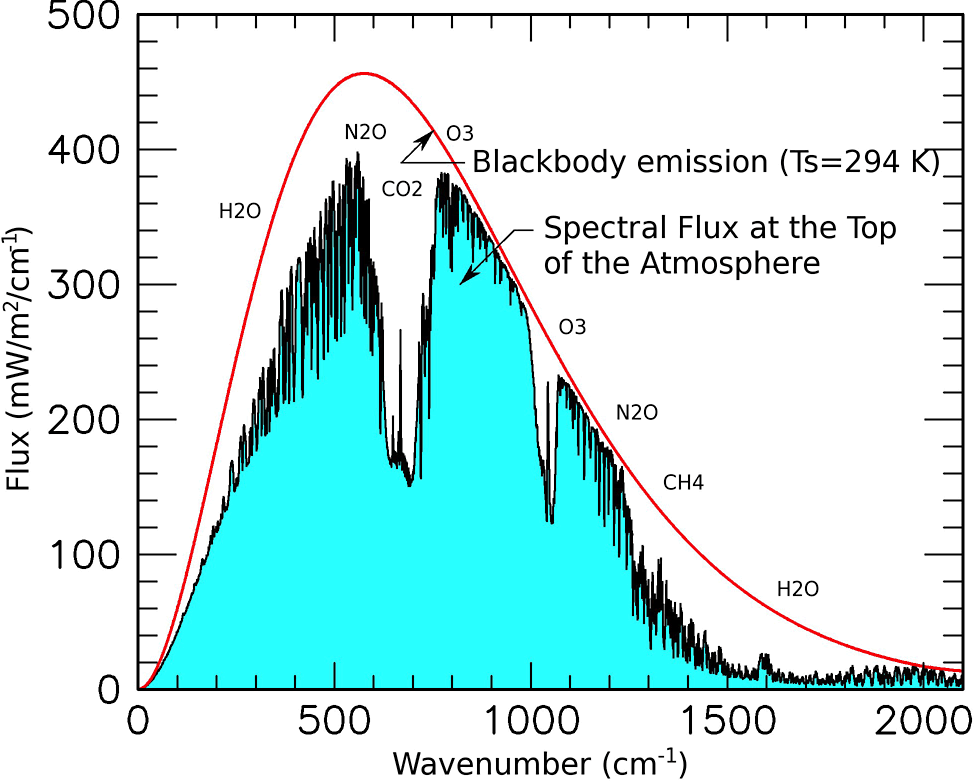Outgoing spectral radiance at the top of Earth’s atmosphere showing the absorption at specific frequencies and the principle absorber. For comparison, the red curve shows the flux from a classic “blackbody” at 294°K (≈21°C ≈ 69.5°F) [From G. Schmidt NASA GISS 2010]

## How thermal emissions scale with temperature

We know that total black body emissions scale as the fourth power of temperature. That total involves an integration over all frequencies. But, how do emissions scale when we look at a particular frequency? That’s plotted below.

When we look at individual frequencies, blackbody emissions scale slower than T4 at lower frequencies and faster than T4 at higher frequencies.

In the vicinity of the carbon dioxide 15-micron / 670 cm-1 absorption/emission band, scaling is quite close to T4. However, when looking at absorption/emission associated with other gases (like water vapor), the temperature scaling may deviate significantly from T4.

## Thermal emissions spectrum vs. altitude / temperature

The following plot shows how the thermal emission spectrum varies with altitude / temperature, assuming the International Standard Atmosphere temperature profile.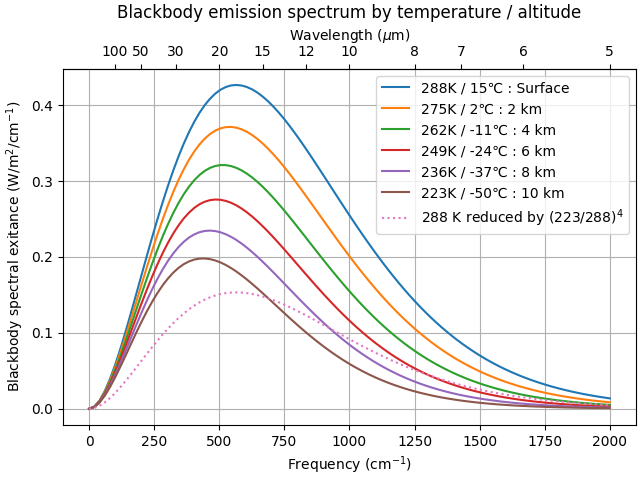[Chart 221108-BBEvFTA-a] Blackbody emission spectrum vs. frequency (wavenumber) for various altitudes, assuming International Standard Atmosphere temperature profile.

The dotted curve shows what the bottom 223K curve would look like if all wavelengths simply scaled as T4. This confirms the result that low frequencies scale with temperature more slowly than this and high frequencies scale more rapidly than this.

## Density and pressure versus altitude

The thermal radiation propagation equations require knowing density,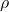, and temperature,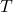, as a function of altitude. For current purposes, I assume temperature decreases linearly in accordance with a fixed lapse rate: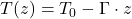, where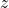is altitude, and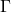is the lapse rate.

We know that pressure, density, and temperature are related by the ideal gas law: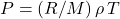, where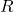is the gas constant and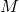is the molar mass of air.

In this section, I consider three models:

• Temperature Power Law (TPL):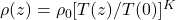where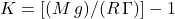, and where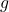is Earth’s gravitational constant. I believe this to be an exact solution to the hydrostatic balance equation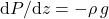when temperature decreases in accordance with a fixed lapse rate. (For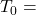288.185 K and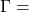6.5℃/km, as in the ISA, the exponent is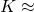4.256.)
• Density Scale Height (DSH):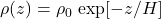where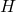is the scale height. (This formula is particularly convenient because it makes it easy to integrate density with respect to altitude.)
• Pressure Scale Height (PSH):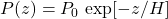whereis the scale height. Wikipedia suggests that this model is a decent fit for Earth with a scale height of about 8.5 km.

In the charts below, I’ve plotted density and pressure as described by these various models.